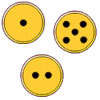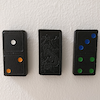#### You may also like### Buzzy Bee

Buzzy Bee was building a honeycomb. She decorated the honeycomb with a pattern using numbers. Can you discover Buzzy's pattern and fill in the empty cells for her?### Fair Exchange

In your bank, you have three types of coins. The number of spots shows how much they are worth. Can you choose coins to exchange with the groups given to make the same total?### Missing Middles

Can you work out the domino pieces which would go in the middle in each case to complete the pattern of these eight sets of three dominoes?

# Twizzle's Journey

##### Age 5 to 7Challenge Level

We had good solutions to this problem from several of you including Mollie who goes to St.Michael's C.of.E Primary School, Adam from Brayton College and Ruth from Swanbourne House School. Ruth said:

First we worked out which route was shortest:
Route $1$ has $22$ squares. Each square takes half an hour, so the total time will be $11$ hours.
Route $2$ has $19$ squares, so it will take $9\frac{1}{2}$ hours.
Route $3$ has $25$ squares, so it will take $12\frac{1}{2}$ hours.
So Route $2$ is the shortest. In a journey of $9\frac{1}{2}$ hours, there will need to be three stops. Each stop takes half an hour, so $3$ half hours = $1\frac{1}{2}$ hours.
This means the total journey time will be $9\frac{1}{2}$ hours $+ 1\frac{1}{2}$ hours $= 11$ hours.

Well thought out, Ruth.